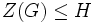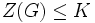# Local powering-invariant subgroup containing the center is intermediately powering-invariant in nilpotent group

## Statement

Suppose$G$ is a nilpotent group and$H$ is a subgroup containing the center of$G$ that is also a local powering-invariant subgroup of$G$. Then,$H$ is an intermediately powering-invariant subgroup of$G$. Explicitly, suppose$K$ is a subgroup of$G$ containing$H$. Then,$H$ is a powering-invariant subgroup of$K$.

## Facts used

1. Torsion-freeness for a prime is subgroup-closed
2. Equivalence of definitions of nilpotent group that is torsion-free for a set of primes

## Proof

Given: A nilpotent group$G$, a subgroup$H$ of$G$ that is local powering-invariant and such that$Z(G) \le H$ where$Z(G)$ is the center of$G$. A subgroup$K$ of$G$ containing$H$. A prime number$p$ such that$K$ is$p$-powered. An element$h \in H$.

To prove: There exists a unique element$x \in H$ such that$x^p = h$.

Proof:

Step no. Assertion/construction Facts used Given data used Previous steps used Explanation
1$Z(G) \le K$.$Z(G) \le H, H \le K$. given-direct
2$K$ is$p$-torsion-free.$K$ is$p$-powered (we are using the powering-injectivity here) given-direct
3$Z(G)$ is$p$-torsion-free. Fact (1) Steps (1), (2) Step-fact combination direct
4 The map$t \mapsto t^p$ is injective in$G$. Fact (2)$G$ is nilpotent Step (3) Step-fact combination direct (specifically, we want to use the implication from (4) to (1) in the multi-part equivalence of Fact (2))
5 There exists an element$x \in K$ satisfying$x^p = h$.$K$ is$p$-powered (we are using the$p$-divisibility here),$h \in H \le K$ given-direct
6 The element$x \in K$ of the preceding step is the unique$p^{th}$ root of$h$ in all of$G$. Steps (4), (5) Step-combination direct
7 The element$x$ of Step (5) is in$H$.$H$ is local powering-invariant in$G$. Step (6) Step-given combination direct.